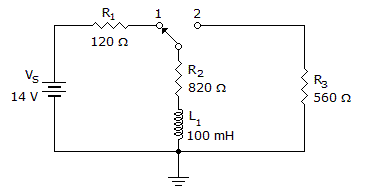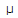# Electronics - Inductors

Exercise : Inductors - General Questions
1.
Electricity may be generated by a wire:
carrying current
wrapped as a coil
that has neutral domains
passing through a flux field
Explanation:
No answer description is available. Let's discuss.

2.In the given circuit, what will the voltage be across R3 25s after the switch is moved to position 2?

2.88 V
5.9 V
8.34 V
14 V
Explanation:
No answer description is available. Let's discuss.

3.
The electrical energy consumed by a coil is stored in the form of:
an electrical field
a force field
an electrostatic field
a magnetic field
Explanation:
No answer description is available. Let's discuss.

4.
What is magnetic flux?
the number of lines of force in webers
the number of lines of force in maxwells
the number of lines of force in teslas
the number of lines of force in flux density